# GSEB Solutions Class 12 Maths Chapter 6 Application of Derivatives Ex 6.1

Gujarat Board GSEB Textbook Solutions Class 12 Maths Chapter 6 Application of Derivatives Ex 6.1 Textbook Questions and Answers.

## Gujarat Board Textbook Solutions Class 12 Maths Chapter 6 Application of Derivatives Ex 6.1Question 1.
Find the rate of change of the area of a circle with respect to its radius, when
(a) r = 3 cm (b) r = 4 cm
Solution:
Let A be the area of the circle of radius r.
∴ A = πr2
and $$\frac{d A}{d r}$$ = 2πr
(a) When r = 3 cm, $$\frac{d A}{d r}$$ = 2π × 3 = 6π cm2
(b) When r = 4 cm, $$\frac{d A}{d r}$$ = 2π × 4 = 8π cm2

Question 2.
The volume of a cube is increasing at the rate of 8 cm³/sec. How fast is the surface area increasing, when the length of an edge is 12 cm?
Solution:
Let x be the length of the cube.
Volume V = x3, Surface area S = 6x2.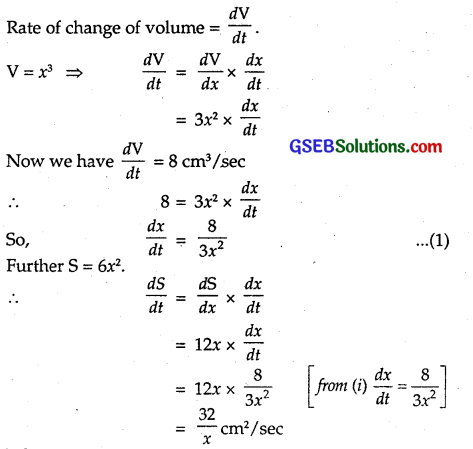When x = 12 cm,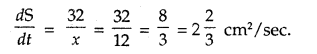Question 3.
The radius of a circle is increasing uniformly at the rate of 3 cm per second. Find the rate at which the area of the circle is increasing, when the radius is 10 cm.
Solution:
Let r be the radius of the circle.
∴ Rate of increase of radius = $$\frac{d r}{d t}$$ = 3 cm/sec.
Now, area of circle π r2 = A (say).
∴ $$\frac{d A}{d t}$$ = $$\frac{d}{d t}$$(πr2) = $$\frac{d}{d t}$$(πr2). $$\frac{d r}{d t}$$
= 2πr × 3 = 6πr
When r = 10,
the rate of change of area = 6π × 10 = 60 π cm2/sec.

Question 4.
An edge of a variable cube is increasing at the rate of 3 cm per second. How fast is the volume of the cube increasing, when the edge is 10 cm long?
Solution:
Let the edge of the cube = x cm.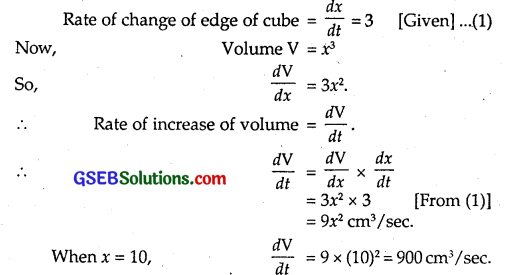Question 5.
A stone is dropped into a quiet lake and waves move in circles at the rate of 5 cm/sec. At the instant, when radius of the circular wave is 8 cm, how fast is the enclosed area increasing ?
Solution:
Let r be the radius of a wave circle.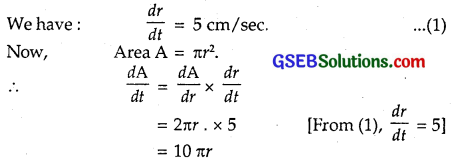When r = 8,
rate of increase in area = $$\frac{d A}{d t}$$
= 10 πr = 10 × π × 8 = 80 π cm2/sec.Question 6.
The radius of a circle is increasing at 0.7 cm/s. What is the rate of increase of its circumference ?
Solution:
The rate of change of radius of the circle w.r.t. time t is given to be 0.7 cm/sec, i.e., $$\frac{d r}{d t}$$ = 0.7 cm/sec.
Now, circumference of the circle is C = 2πr.
∴ The rate of change of circumference w.r.t. t is given by
= $$\frac{d \mathrm{C}}{d t}$$ = [$$\frac{d}{d r}$$(2r) . $$\frac{d r}{d t}$$]
= 2π . $$\frac{d r}{d t}$$ = 2π × 0.7
= 1.47π cm/sec.

Question 7.
The length x of a rectangle is decreasing at the rate of 5 cm/minute and the width y is increasing at the rate of 4 cm/ minute. When x = 8 cm, then y = 6 cm. Find the rate of change of
(a) the perimeter and
(b) the area of the rectangle.
Solution:
(a) The length x of a rectangle is decreasing at the rate of 5 cm/minute.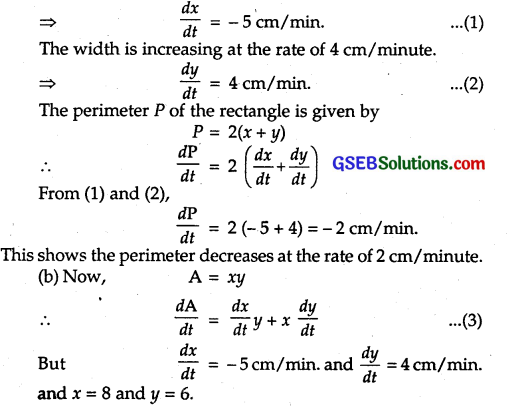∴ Putting these values in (3), we get
$$\frac{d \mathrm{~A}}{d t}$$ = (- 5 × 6) + 8 × 4 = – 30 + 32
= 2cm2/min.
⇒ A increases at the rate of 2 cm2/minute.

Question 8.
A balloon which always remains spherical is being inflated by pumping in 900 cubic centimetres of gas per second. Find the rate at which the radius of the balloon increases, when the radius is 15 cm.
Solution:
Volume V of the spherical balloon with radius r is given by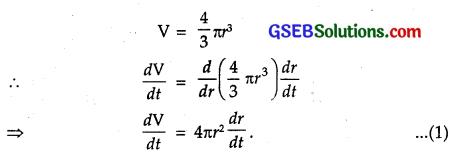It is given that the balloon is inflated by pumping in 900 cubic cm of gas per sec.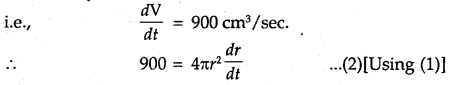When radius is 15 cm, putting r = 15 in (2), we have :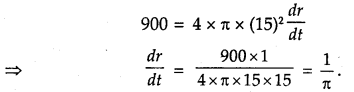Hence, the radius of the balloon is increasing at the rate of $$\frac{1}{\pi}$$ cm/sec.Question 9.
A balloon which always remains spherical has a variable radius. Find the rate at which its volume is increasing with the radius, when the latter is 10 cm.
Solution:
Let r be the variable radius of the balloon, which is in the form of a sphere.
So, volume of the sphere i.e., V = $$\frac{1}{2}$$ πr3.
Rate of change of volume w.r.t. r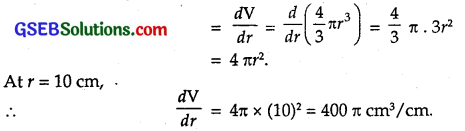Question 10.
A ladder 5 m long is leaning against a wall. The bottom of the ladder is pulled along the ground, away from the wall, at the rate of 2 cm/s. How fast is its height on the wall decreasing, when the foot of the ladder is 4 m away from the wall?
Solution:
Let AB be the ladder and OB be the wall.
At an instant, let
OA = x, OB = y.
∴ x2 + y2 = 25
On differentiating w.r.t. t, we get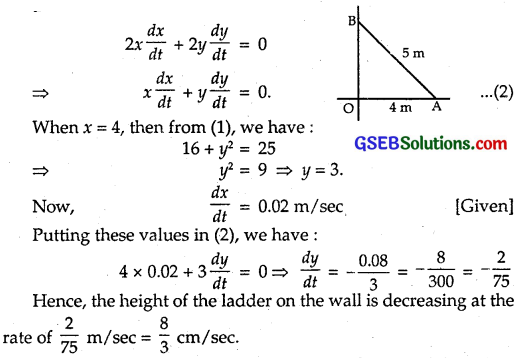Hence, the height of the ladder on the wall is decreasing at the rate of $$\frac{2}{75}$$ m/sec = $$\frac{8}{3}$$ cm/sec.Question 11.
A particle moves along the curve 6y = y3 + 2. Find the points on the curve at which the y-coordinate is changing 8 times as fast as the coordinate.
Solution:
We have : 6y = x3 + 2
Differentiating (1) w.r.t. t, we get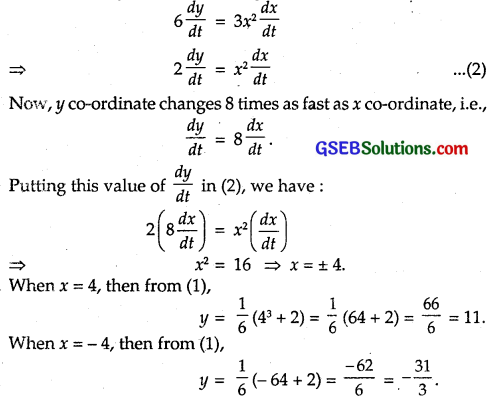Hence, the required points are (4,11) and (-4, –$$\frac{31}{3}$$).

Question 12.
The radius of an air bubble is increasing at the rate of $$\frac{1}{2}$$ cm per second. At what rate is the volume of the bubble increasing, when the radius is 1 cm?
Solution:
Let r be the radius of the air bubble. If V is the volume of bubble at time f, then V = $$\frac{4}{3}$$ πr3.
The rate of increase of radius w.r.t. time t
i.e., $$\frac{d r}{d t}$$ = —$$\frac{1}{2}$$ cm/sec. …(1) [Given]
Now, the rate of increase of volume V w.r.t. time t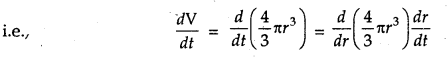$$\frac{4}{3}$$ π. 3r2 . $$\frac{d r}{d t}$$
– 4 πr2 ($$\frac{1}{2}$$)
= 2πr2
Hence, the rate of increase of volume when radius is 1 cm
= 2π . 12 = 2π cm3/sec.

Question 13.
A balloon which always remains spherical has a variable diameter $$\frac{3}{2}$$(2x + 1). Find the rate of change of its volume with respect to x.
Solution:
Diameter of the sphere = $$\frac{3}{2}$$(2x + 1).
Radius of the sphere = $$\frac{3}{2}$$(2x + 1).
Volume V of the sphere is given by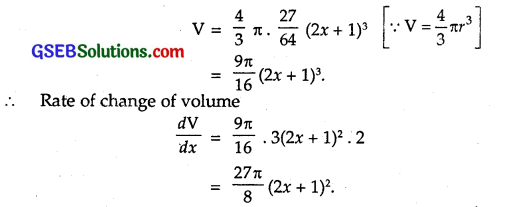Question 14.
Sand is pouring from a pipe at the rate of 12 cm³/sec. The falling sand forms a cone on the ground in such a way that the height of the cone is always one-sixth of the radius of the base. How fast is the height of the sand-cone increasing, when the height is 4 cm?
Solution:
Let r and h be the radius and height of the sand-cone at time t respectively.
So, h = $$\frac{r}{6}$$ …….. (1)
Let V be the volume of the cone.
So, V = $$\frac{1}{2}$$ πr2h = $$\frac{1}{3}$$ π(6h)2h [∵ r = 6h]
= $$\frac{1}{3}$$ π × 36h2 . h = 12 πh3
∴ $$\frac{d \mathrm{~V}}{d t}$$ = 12 π (3h2) $$\frac{d h}{d t}$$ = 36πh2 $$\frac{d h}{d t}$$ ………. (2)
It is given that sand is pouring at the rate of 12 cm3/sec.
∴ $$\frac{d \mathrm{~V}}{d t}$$ = 12 …………… (3)
From (2) and (3), we have :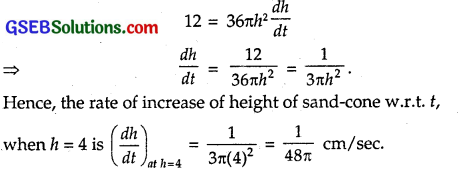Question 15.
The total cost C(x) associated with production of x units of an item is given by C(x) = 0.007 x3 – 0.003x2 + 15x + 4000. Find the marginal cost, when 17 units are produced.
Solution:
Marginal cost MC = Instantaneous rate of change of total cost at any level of output
= $$\frac{d \mathrm{C}}{d x}$$
Now, C(x) = 0.007x3 – 0.003 x2 + 15x + 4000
∴ MC = $$\frac{d \mathrm{C}}{d x}$$ = 3 × 0.007x2 – 2 × 0.003x + 15 dx
= 0.021 x2 – 0.006x + 15
When x = 17, $$\frac{d \mathrm{C}}{d x}$$ = 0.021 × 17 × 17 – 0.006 × 17 + 15
= 6.069 – 0.102 + 15 = 20.967.
∴ Marginal Cost = ₹ 20.97.Question 16.
The total revenue received from the sale of x units of a product is given by R(x) = 13x2 + 26x + 15. Find the marginal revenue, when x = 7.
Solution:
Marginal Revenue (MR) = Rate of change of total revenue with respect to the number of items sold at an instant = $$\frac{d \mathrm{R}}{d x}$$.
We know that R(x) = 13x2 + 26x + 15
∴ MR = $$\frac{d \mathrm{R}}{d x}$$ = 26x + 26 = 26 (x + 1)
When x = 7, ∴ MR = 26 (x + 1) = 26 (7 + 1) = 26 × 8 = 208.
Hence, Marginal Revenue = ₹ 208.

Question 17.
The rate of change of area of a circle with respect to its radius r at r = 6 cm is
(A) 10π
(B) 12π
(C) 8π
(D) 11π
Solution:
Area of circle (A) = πr2.
∴ The rate of change of area w.r.t. its radius r = $$\frac{d \mathrm{A}}{d r}$$ = 2πr
At r = 6, $$\frac{d \mathrm{~A}}{d r}$$ = 2π × 6 = 12π cm2/radius.
⇒ Part (B) is the correct answer.Question 18.
The total revenue (in Rupees) received from the sale of x units of a product is given by R(x) = 3x2 + 36x + 5. The marginal revenue at x = 15 is
(A) 116
(B) 96
(C) 90
(D) 126
Solution:
R(x) = 3x2 + 36x + 5
∴ MR = $$\frac{d \mathrm{~R}}{d x}$$ = 6x + 36
∴ For x = 15, $$\frac{d \mathrm{~R}}{d x}$$ = 6 × 15 + 36
= 90 + 36 = 126 [Given x = 15]
⇒ Part (D) is the correct answer.